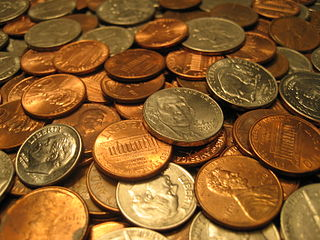# Correct Change I

You are given a certain number of `nickels` and `pennies` and a desired `sum` of cents. Is it possible to select the exact change to reach that sum?

You will be given three numbers as input - `nickels`, `pennies` and `sum`. Return `true` if you can select coins to reach `sum` exactly, and `false` otherwise.

In this challenge, you can be given any number of `nickels` but only 0 to 4 `pennies`.Image from Wikipedia

Note: A nickel is worth 5 cents. A penny is worth 1 cent.

Input/Output Format
Boilerplate code is provided for Java. For other languages, read in the numbers from the standard input in the following format.

• The input starts with N, the number of cases.
• N lines follow, which each contains 3 integers in order for the number of `nickels`, number of `pennies`, and the desired `sum`.

Print out true or false to the output for each case on its own line.

Click on "View Raw I/O" below to view sample input and output.

### Challenge

Can you get the exact sum using the given number of nickels and pennies?

Alternatively, you can try out Learneroo before signing up.

•#### Hongwen

For input set 5 3 19, we can get 19 using 3 pennies and 4 nickels. So it should be true there.

•#### Hongwen

Sorry, I mistook nickels and pennies...

•#### Amboss

5, 4, 23 will result false !!!!!! it ca't be true 5*5 +4 = 24 ?????????????????

•#### Learneroo

@Amboss, the challenge is to see if you can select the exact change from the coins you are given. So if you have 5 nickels, you can select 4 of them, add 4 pennies for a sum of 24 cents.

•#### Кирилл Варивода

I've made the code, but when I run table below shows that all cases are correct EXCEPT that "Your Output" is incorrect, it's red.
I don't know why, I checked some made up cases manually on Eclipse.

•#### Learneroo

Кирилл, When given 7 nickels and 3 pennies, can you get 35 cents? Yes, you just select the nickels and ignore the pennies. Check your code to see what it returns.

•#### Кирилл Варивода

Admin (?), I've make some changes, even few, but still, there is something wrong. Your example works, thanks for it!)
I'm just curious where I get lost.

•#### Learneroo

Кирилл. there's another example you're getting wrong. I think you should approach this problem a little differently. You can solve it with one if-else statement.

•#### Кирилл Варивода

You can solve it with one if-else statement.
You're right. And only now it's shows "Correct". I have to say that with no mathematical help I won't do that. So, on my opinion this problem more related to mathematics rather then to programming.

•#### David

OMG :( this took me forever also my code is extensive.... looks like complying with DRY is going to take me looong time to master.

•#### saeed

At the end of my program I write << return boolean; >> but program say error:

variable bool might not have been initialized
return bool;

•#### saeed

I found my solution;
when I defined boolean bool I must give amount to it LIKE boolean bool = false;

•#### Learneroo

I archived many comments here with solutions. You can link to your solution (in your submissions) to share it, but you shouldn't paste the whole solution in here.

•#### Learneroo

•#### Shena

i'm confused i'm not familiar with nickel and pennies and cents -.- do they have relationships with each other ? like how many cents are there in pennies or in nickel ? :)

•#### Brayden Plush

American Currency^ LOL

•#### Calvin

For those of you in different countries: Pennies are 1 cent, Nickles are 5 cents (each).

•#### Learneroo

•#### thales

I think i have a good solution? is it a smart solution?

my code

•#### Learneroo

•#### Ravi

I wrote my code in python. it's working if i run this in some other compiler but here it's not.
my code

•#### Learneroo

Boilerplate code for this challenge wasn't provided for Python, so you need to take in the input yourself from STDIN.

•#### Lukas

``````    int nCount = sum / 5;
if (nCount <= nickels) {
return sum % 5 <= pennies;
} else {
return false;
}
``````
•#### Prateek

int a=sum/5;
int b=sum%5;
if(nickels>=a && pennies>=b){
return true;
}
return false;

•#### Charlotte

``````if ((sum%nickels) > pennies) return false;
return true;
``````

how the heck did this pass all the tests..

•#### Learneroo

Thanks, that's actually an edge case that wasn't covered before. The last test case will now check that condition so that code no longer passes.

•#### Amanpreet Singh

I did this way
if(nickels>=sum/5 && pennies>=sum%5)
{
return true;
}
else
{
return false;
}

•#### Kyle Lemon

Here's some working boilerplate for Ruby:

n = gets.chomp.to_i
n.times do
input = gets.chomp.split(" ").map(&:to_i)
nickels = input
pennies = input
desired_sum = input
print correct_change?(nickels, pennies, desired_sum), "\n"
end

•#### Fede

here goes my take!

if (sum % nickels <= pennies && ((nickels *5)+ pennies) >= sum){
return true;
}
else{
return false;

•#### Soner Gönül

Here is mine;

``````    public static bool doStuff(int nickels, int pennies, int sum)
{
if (nickels >= sum / 5)
{
return pennies >= sum - sum / 5 * 5;
}

return false;
}
``````
•#### AMR

I am stuck on this

Quick question - Can this exercise be done only with if statements?

Lang - Python

Appreciate the help

Thank you

•#### Roger

function recipe(sum ,nickels, pennies) {
let nickel = 5 * nickels / 100
let penny = 1 * pennies / 100
let change = nickel + penny
if (penny <= 4 && change % sum === 0) {
return 'true'
} else if (penny > 4) {
return 'false'
} else {
return 'false'
}
};